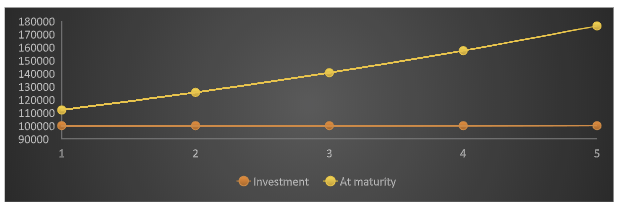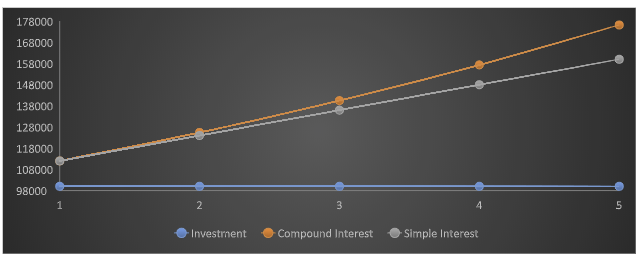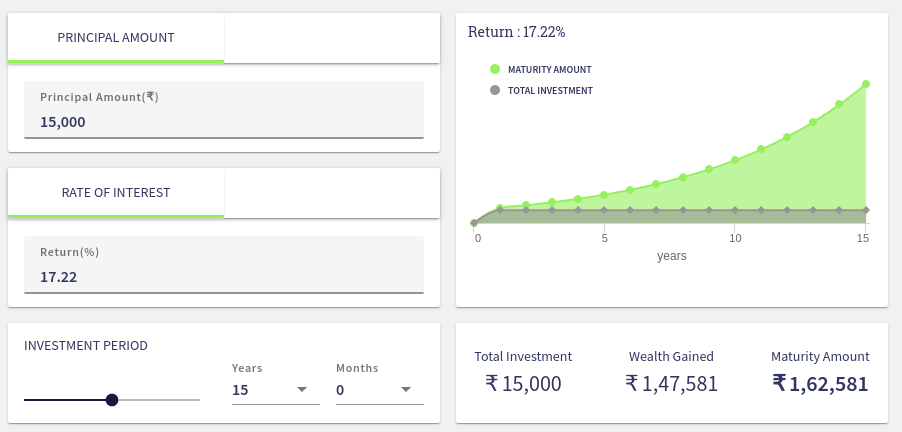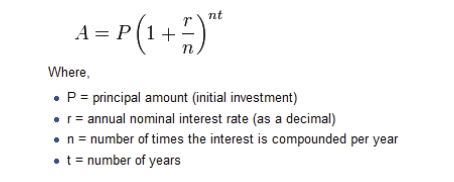# Compound Interest Magic Formula

## Compound Interest Calculator

Compound interest in simple terms means the interest on interest. When the principal includes the accumulated interest of the previous periods and interest is calculated on this then they say it’s compound interest. Compounding is done on loans, deposits, and investments. The frequency of compounding is basically the number of times the interest is calculated in a year. Daily, weekly, monthly, quarterly, half-yearly, and annually are the most common compounding frequencies. The higher the frequency of compounding, the greater the amount of compound interest. The frequency of compounding depends on the instrument. A credit card loan is usually compounded monthly and a savings bank account is compounded daily.

Albert Einstein rightly said “Compound interest is the 8th wonder of the world. He who understands it earns it and he who doesn’t pays it.” Compounding is a very powerful concept. This is because the interest of your invested money is also earning interest. The value of the investment keeps growing at a geometric rate (always increasing) than at an arithmetic rate (straight-line). Your money keeps on multiplying over a period of time. Also, if paying interest is ignored or if there is any delay in paying the loan then the interest burden will surely be high. Also, to take advantage of compounding one has to increase their frequency of loan payments. This way they can pay lesser interest than what they are liable to pay.

Investing in mutual funds is one of the easiest ways of reaping the benefits of compounding. Opting to reinvest dividends or choosing a growth plan results in purchasing more shares of the fund. More interest accumulates over time through continuous purchasing and the investment will grow in value.

## How to calculate compound interest?

Compound interest can be calculated with a simple formula.

Compound Interest = Total amount of Principal and Interest in future (or Future Value) less Principal amount at present (or Present Value)

Compound Interest = P [(1 + i) n – 1]

P is principal, I is interest rate, n is number of compounding periods.

An investment of Rs 1,00,000 for 5 years at 12% rate of return compounded annually is worth Rs 1,76,234. From the graph below we can clearly see how an investment of Rs 1,00,000 has grown in 5 years.In compound interest one earns interest on interest. Therefore, the investment already includes all the previous interests. And interest is paid on that.

Year Investment(Rs) Interest(Rs) At maturity(Rs)
1 1,00,000 12,000 1,12,000
2 1,12,000 13,440 1,25,440
3 1,25,440 15,052.8 1,40,492.8
4 1,40,492.8 16,859.14 1,57,351.9
5 1,57,351.9 18,882.23 1,76,234.2

By understanding the importance of compound interest and acting on it by investing in appropriate investments, one can achieve high returns.

## Simple vs compound interest

Having simple interest for loans is very easy as the interest payments are standard. But when it comes to investments one can earn more from compound interest. The basic difference between simple and compound interest is that the interest is not added to the principal in simple interest. To earn interest on interest on has to immediately reinvest the interest earned. But in compounding this happens automatically with no extra effort needed.

Simple interest is calculated with a simple formula which is Principal*interest rate*tenure. The simple interest amount remains the same through the tenure of investment or loan. It is easy to calculate than compound interest.

The return from compounding is higher than that of simple interest.

The interest earned grows rapidly in compound interest and in simple interest, it remains constant.

The principal amount in simple interest remains constant, while in the compound interest the principal amount keeps increasing as the interest from previous periods is added to it.

Also, having a loan in simple interest ensures standard interest payments. But in compounding the interest payment comes down as the principal is being repaid. If not repaid on time the interest burden keeps increasing.

Let’s look at an example of an investment of Rs 1,00,000 invested for 5 years earning an interest of 12% both in simple and compound interest.It can be clearly seen that at maturity the amount from compounding is higher than that from simple interest. The investment value increases at faster pace in compounding. With the same initial investment at the same interest rate for a same tenure the gain from compounding is higher than from simple interest. Even with a complex calculation, compounding is beneficial than simple interest. Compounding is more of a real time concept than simple interest.

## How to use Compound Interest calculator?

Use Upwardly’s Compound Interest calculator to find how much corpus you would earn at the end of your investment period.

Here, all you need to do is enter the principal amount that you would want to invest and the time period. Below is an example, where Rs 1,50,000 invested for 15 years at the rate of 12%. This investment would earn you an interest of Rs 6,71,035.

The Link has been Mentioned in the last.The rate of interest can be changed and you can see how much you would gain at the end of your investment period.

## How to take advantage of compound interest?

We’ve seen how compound interest has a dramatic positive effect on investments. One needs to know how to take advantage of this. Here are a few ways one can take advantage of compound interest.

Investing early and regularly: Invest early. This will ensure that your money is earning at its full potential. Also, investing regularly is as important as investing early. Investing regularly will help in avoiding the timing of the market. By investing small amounts regularly one can accumulate large amounts

Hold your investment for the long term: Holding the investments for long will help earn interest for a longer period. Holding for a long period is very important because compounding works only in the long-term.

Frequency of compounding intervals: The more the frequency of compounding, the larger the interest earned. Choose investments that pay interest more frequently than the ones which pay less frequently.

Higher rates of return: Only a high return investment can earn you more money. Make sure that you pick up investment options with higher rates of return. Like mutual funds. Only then one can reap the full benefits of compounding.

Increase the frequency of loan payments: By increasing the frequency of loan payments one can reduce the interest burden. Try paying one or two months extra EMI’s in a year to reduce the total interest paid on loans taken.

### What is the RULE OF 72 ?

For Example The Rate of Interest in your country is 7% then just divide 72/7. The Answer comes up to 10.28 years. It means if you invest 10000 Rs or X Amount it will be doubled in 10.28 years if the interest you are getting is 7%.## Importance of compounding intervals

The frequency of compounding and wealth accumulation are directly related. The higher the frequency of compounding, the more the accumulation of wealth. Let’s look at the growth of Rs 10,000 at 10% interest compounded at different frequencies.

Time Annual Quarterly Monthly
1 11,000.00 11,038.13 11,047.13
5 16,105.10 16,386.16 16,453.09
10 25,937.42 26,850.64 27,070.41

It is very clear from the above example that the higher the compounding interval, higher is the wealth accumulated. Also, longer the investment tenure higher is the wealth accumulated.

Let the magic of compounding work for you by investing regularly and staying invested for long horizons and increasing the frequency of loan payments. By familiarizing yourself with such concepts you can make better financial decisions and earn higher returns.

FAQs

### Q: What are the frequencies in which compound interest is calculated?

Ans. The compound interest calculator includes options for:

• Daily compounding
• Monthly compounding
• Quarterly compounding
• Half yearly compounding
• Yearly compounding

With savings accounts, interest can be compounded at either the start or the end of the compounding period (month or year).

### Q: What is the effective annual rate?

Ans. The effective annual rate is the rate that actually gets paid after all of the compounding. When compounding of interest takes place, the effective annual rate becomes higher than the overall interest rate. The more times the interest is compounded within the year, the higher the effective annual rate will be.

### Q: Is compound interest online calculator dynamic enough to calculate on fluctuating interest rates?

Ans.Yes, the online interest calculators generally ask you to enter the amount, rate of interest, time period, etc, manually so that you can get dynamic results as per needs.

### Q: What is the formula difference between simple interest and compound interest?

Ans.Here I: interest, P: Principal, R: Rate, T: Time and A: Amount:

Simple interest rate formula is,
I= P*R*T/100; hence A = P + I
Compound interest rate formula is### Q: Why is compound interest better than simple interest?

Ans.In compound interest, the investment grows much faster than the simple interest as the interest is paid on both investment as well as previous interest.

Let’s take an example:
Assume an investment of Rs 1 lakh is made. Let us see what would be the return with an option of simple and compound interest, given the rate of interest is 20% annually for a period of 3 years.
The simple interest earned will be I= P*R*T/100
That is, I = 1,00,000*20*3/100 = Rs 60,000
And in case of compound interest, amount is P (1 + r/n) ^ nt That is, A=1,00,000(1+0.2) ^3 = 1,00,000(1.728) = 1,72,800 Hence, I = A-P i.e. 1,72,800-1,00,000 = Rs 72,800 You can see it yourself that there is a great difference in the returns between the two. Therefore, compound interest proves to be a good option for investment the return is higher than simple interest.

Link to use the Compound Interest Calculator

https://www.upwardly.in/en/compound-interest-calculator

Blog by UPWARDLY

Like, Share, Comment

Rushabh Thakker | TheFineMen